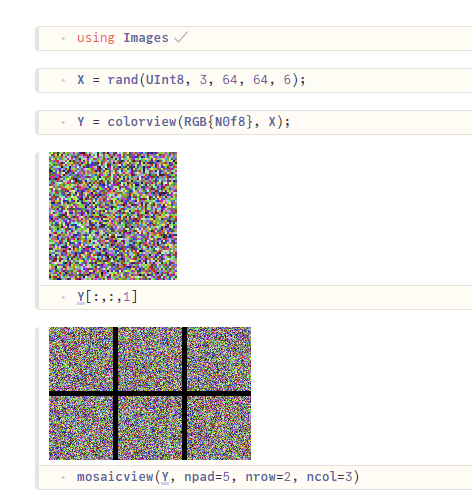# Display image as Array{UInt8, 4}: in julia

I load the dataset from h5 file with HDF5.jl to work with binary classifying problems with neural networks

I want to display an image from

``````train_set_x
3×64×64×209 Array{UInt8, 4}:
``````

Element type of this array is UInt8 but when I show it, it returns as hex but not decimal

I had a look at this answer but the code didn’t work for me. Python seems user-friendly than Julia when display an Image from array

``````plt.imshow(train_set_x_orig[index])
``````

Why array in hex not in decimal? What is the most user-friendly way to display array as an image? Thank you.

You need to convert to colors first. Images.jl has a convenience function `colorview` for that. See Quickstart · JuliaImages

Note that `Y` here, doesn’t make a copy of `X`, but merely reinterprets the original array as colors.
Jupyter, Pluto and VSCode know how to display colors:Edit: Sorry, I’ve missed that you were already using colorview in your code. The point is that you need to provide a number format for RGB, in this case N0f8 is appropriate.

It’s not clear to me if you want it displayed as an image or as a numerical array. If as image, se previous post, if as numerical array, you can just convert to `Int` by typing

``````Int.(train_set_x)
``````

I solved the problem with your suggestion

``````raw_img = train_set_x[:, :, :, :]
img_perm = colorview(RGB{N0f8}, raw_img)
transposed_img = permutedims(img_perm, (2, 1, 3))
# imrotate(img_perm, π/2)|
mosaicview(transposed_img[:, :, 1:20], nrow=4, ncol=5)
``````

The h5 file come from python code and I translate to julia. The dimension order in julia (3, 64, 64) a lil bit different to numpy array (64, 64, 3), I don’t know why Images.jl choose this, but now I learned about permutedims so it’s ok.